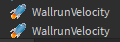# Body velocity going backward instead of forward?

okay so, i made my wallrunning in another game and when i put it on my main game it does not work?
Mainly, the body velocity goes backwards
i tried doing:

``````BodyVelocity.Velocity = Vector3.new(-20,0,0)
``````

Since the original one is:

``````BodyVelocity.Velocity = Vector3.new(20,0,0)
``````

I also tried:

``````BodyVelocity.Velocity = HRP.CFrame.LookVector * 20
``````

and of course, -20

But its not working, any solutions? Thanks

Edit: here is the script where it created the BodyVelocity

``````local bodyVelocity = Instance.new("BodyVelocity")
bodyVelocity.Name = "WallrunVelocity"
bodyVelocity.Parent = HRP
bodyVelocity.Velocity = Vector3.new(20,0,0)
``````
1 Like

I don’t really see any issues here.

1 Like

Hmm, i have noticed that they create body velocity twice, and when bouncing (when body velocity is destroyed it bounces me) it creates it twice aswell, but from the prints i see no problems…

edit:
Here is a screenshot of the body velocity being two:here is the script where it creates the uh velocity

``````local bodyVelocity = Instance.new("BodyVelocity")
bodyVelocity.Name = "WallrunVelocity"
bodyVelocity.Parent = HRP
bodyVelocity.Velocity = Vector3.new(20,0,0)
``````

Edit: since the bounce velocity, also makes me bounce twice and has a problem aswell here is the script where it creates the bounce script:

``````	if bool == true then
local BodyVelocity = Instance.new('BodyVelocity')
BodyVelocity.MaxForce = Vector3.new(40000,40000,40000)

BodyVelocity.Velocity = -HRP.CFrame.rightVector * 40 + Vector3.new(0,50,0)
BodyVelocity.Parent = HRP